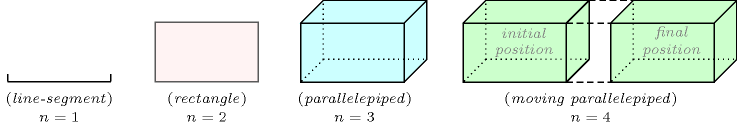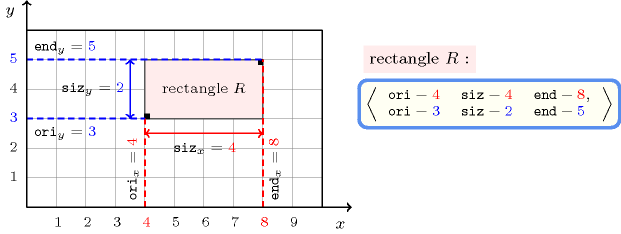### 3.7.175. Orthotope

##### Figure 3.7.46. Illustration of the notion of orthotope for various number of dimensions $n$A constraint involving orthotopes. An orthotope corresponds to the generalisation of the rectangle and box to the $n$-dimensional case. In addition its sides are parallel to the axes of the  placement space. Figure 3.7.46 illustrates the notion of orthotope for $n=1,2,3$ and 4. A collection usually named $\mathrm{𝙾𝚁𝚃𝙷𝙾𝚃𝙾𝙿𝙴}$, declared as $\mathrm{𝙾𝚁𝚃𝙷𝙾𝚃𝙾𝙿𝙴}-\mathrm{𝚌𝚘𝚕𝚕𝚎𝚌𝚝𝚒𝚘𝚗}\left(\mathrm{𝚘𝚛𝚒}-\mathrm{𝚍𝚟𝚊𝚛},\mathrm{𝚜𝚒𝚣}-\mathrm{𝚍𝚟𝚊𝚛},\mathrm{𝚎𝚗𝚍}-\mathrm{𝚍𝚟𝚊𝚛}\right)$, defines for each dimension $d$ (with $d\in \left[1,n\right]$) the coordinate of its lower corner, the size and the coordinate of its upper corner in dimension $d$. Figure 3.7.47 illustrates the representation of an orthotope for $n=2$.

##### Figure 3.7.47. Representation of an orthotope when the number of dimensions $n=2$ in term of the collection $\mathrm{𝙾𝚁𝚃𝙷𝙾𝚃𝙾𝙿𝙴}-\mathrm{𝚌𝚘𝚕𝚕𝚎𝚌𝚝𝚒𝚘𝚗}\left(\mathrm{𝚘𝚛𝚒}-\mathrm{𝚍𝚟𝚊𝚛},\mathrm{𝚜𝚒𝚣}-\mathrm{𝚍𝚟𝚊𝚛},\mathrm{𝚎𝚗𝚍}-\mathrm{𝚍𝚟𝚊𝚛}\right)$